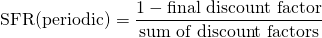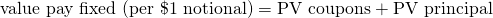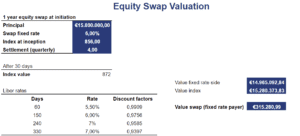# Equity Swap Valuation

To price an N-period pay-fixed equity swap, we use the same formulas as we used to value an interest rate swap. In particular, we first need to calculate the appropriate set of discount factors. Once we have the discount factors, we can determine the swap fixed rate. To value the pay-fixed equity swap, we need to use a different formula, which we will discuss in more detail below. Thus, equity swap valuation can easily be accomplished using two formulas.

On this page, we discuss how to price and value a pay-fixed equity swap. We also provide numerical example that is implemented using Excel. The equity swap valuation calculator is available for download at the bottom of the page.

## Equity Swap pricing

Let’s first discuss how to determine the swap fixed price of an equity swap. As we mentioned in the introduction, we can use the exact same formula as the one that is used to price a interest rate swap to determine the swap fixed rate. In particular, we use the following formula.For more details on this formula, the page on interest rate swaps explains how to apply the formula.

## Equity swap valuation formula

Let’s turn to the equity swap valuation model. The following formula should be used to value an existing pay fixed equity swapLet’s consider an equity swap example to make things a bit more concrete.

## Equity swap valuation example

Let’s apply the above formula to value an existing pay-fixed, receive-equity-returns swap. The following table illustrates the necessary calculations. The equity swap value calculator can be downloaded at the bottom of the page.## Summary

We discussed the valuation of equity swaps. In that case of a pay-fixed equity swap, we can use the formulas for a standard interest rate swap.